Courses

# Notes | EduRev

## NEET : Notes | EduRev

The document Notes | EduRev is a part of the NEET Course Physics 28 Years Past year papers for NEET/AIPMT Class 12.
All you need of NEET at this link: NEET

Q.1. The electrostatic force between the metal plates of an isolated parallel plate capacitor C having a charge Q and area A, is :-     (2018)
A: independent of the distance between the plates.
B: linearly proportional to the distance between the plates
C: proportional to the square root of the distance between the plates.
D: inversely proportional to the distance between the plates.
Ans:
A
Solution:

F =  QE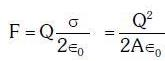∴ Electrostatic force is independent of distance between plates

Q.2. A capacitor is charged by a battery. The battery is removed and another identical uncharged capacitor is connected in parallel. The total electrostatic energy of resulting system :-   (2017)
A: Decreases by a factor of 2
B: Remains the same
C: Increases by a factor of 2
D: Increases by a factor of 4
Ans: A
Solution: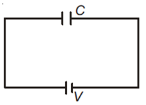Charge on capacitor
q = CV
when it is connected with another uncharged capacitor.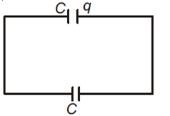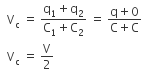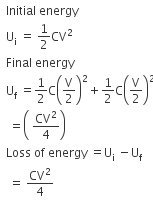Q.3. The diagrams below show regions of equipotentials:-     (2017)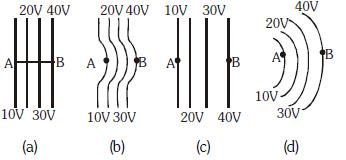A positive charge is moved from A to B in each diagram.
A: Maximum work is required to move q in figure (c)
B: Minimum work is required to move q in figure (a)
C: Maximum work is required to move q in figure (b)
D: In all the four cases the work done is the same
Ans:
D
Solution:
W = qΔV
as ΔV is same in all conditions, work will be same.

Q.4. A capacitor of 21F is charged as shown in the diagram. When the switch S is turned to position 2, the percentage of its stored energy dissipated is :    (2016)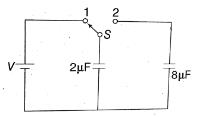A: 80%
B: 0%
C: 20%
D: 75%
Ans:
A
Solution:

Consider the figure given above.
When switch S is connected to point 1, then initial energy stored in the capacitor is given as,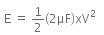When the switch S is connected to point 2, energy dissipated on connection across 8μF will be,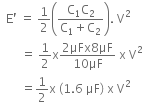Therefore, per centage loss of energy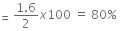Q.5. A parallel plate air capacitor of capacitance C is connected to a cell of emf V and then disconnected from it. A dielectric slab of dielectric constant K, which can just fill the air gap of the capacitor, is now inserted in it. Which of the following is incorrect ?     (2015)
A: The charge on the capacitor is not conserved.
B: The potential difference between the plates decreases K times
C: The energy stored in the capacitor decreases K times

D: the change in energy stored is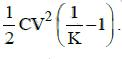Ans:
A
Solution:
A parallel plate air capacitor of capacitance C is connected to a cell of emf V and then disconnected from it.
The charge on the capacitor is given by
Q = CV
The energy stored in the capacitor is
E = 1/2 CV2
When a dielectric slab of dielectric constant K is inserted in it, the charge Q is conserved.
The capacitance becomes K times the original capacitance. (C' = KC)
The voltage becomes 1/K time the original voltage
V' = V/K
The change in energy stored is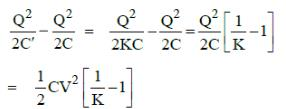Q.6. In a region, the potential is represented by V(x, y, z) = 6x − 8xy − 8y + 6yz, where V is in volts and x, y, z are in meters. The electric force experienced by a charge of 2 coulomb situated at point (1,1,1) is:     (2014)
A: 24N
B: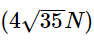C: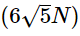D: 30N
Ans:
B
Solution: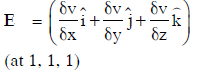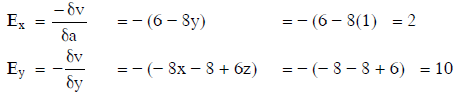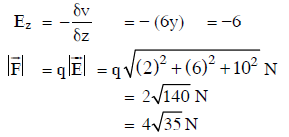Q.7. A conducting sphere of radius R is given a charge Q. The electric potential and the electric field at the centre of the sphere respectively are :    (2014)
A: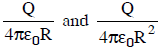B: Both are zero
C: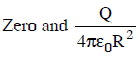D: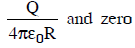Ans:
D
Solution: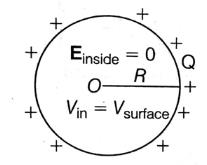Electric field inside, Einside = 0
Potential, Vinside = Vsurface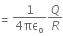Offer running on EduRev: Apply code STAYHOME200 to get INR 200 off on our premium plan EduRev Infinity!

14 docs|25 tests

,

,

,

,

,

,

,

,

,

,

,

,

,

,

,

,

,

,

,

,

,

;